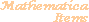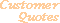Home Older Mathematica Items

Scientific Arts

# From Simple Tabular Formatting to Automatic Report Generation

## Formatting a Simple Table

Let us say that we want produce a tabular report on the analysis of several sets of data contained in columnar format in an external file. The data are in the file file.dat. We first import the data. (If file.dat is not placed in a directory that is contained in Mathematica's \$Path variable, you can make it available by executing the function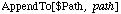where path is the path to the needed directory.)

``````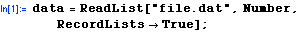``````

Since the data are in columns and the ReadList function returns a list of rows we can access the data by transposing the matrix of data.

``````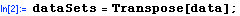``````

Load in the standard package Statistics`DescriptiveStatistics`.

``````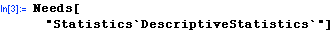``````

This package contains functions that give various statistical properties of a data set. Here we want to compute the first four moments of the data: the mean, variance, and skewness. To do this efficiently we write a small function that does it all at once.

``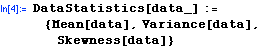``

The following then gives us a list of the desired statistics for each of the data sets. The function Map effectively applies the DataStatistics function to each of the data sublists.

``````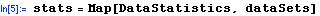``````
`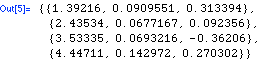`

These results can be presented as a table using the formatting command TableForm.

``````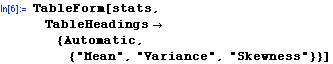``````
 Out=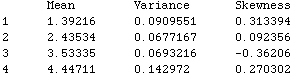Considerably more control can be exercised by using a GridBox. There are many approaches to this, here is an example. First we wrap GridBox around stats and format it by using DisplayForm.

``````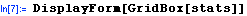``````
 Out=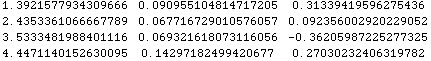By using options we can modify this to add a frame and column lines.

``````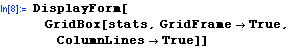``````
 Out=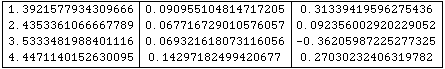In the following we reduce the number of digits in the displayed numbers and add color to the column headings.

``````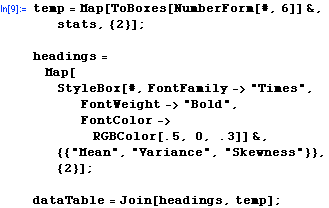``````
``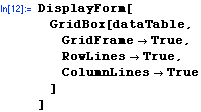``
 Out=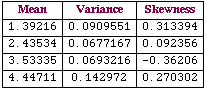The following prints a formatted cell. The visual result is the same as above, but note that the cell that is returned does not have a cell label.

``````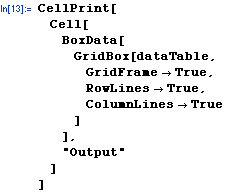``````Here we use CellPrint to print three cells in sequence (the individual cells are contained as the elements of the list that is the argument of CellPrint). The three cells that follow this command are the result of executing it. Thus we are essentially writing a small report.

``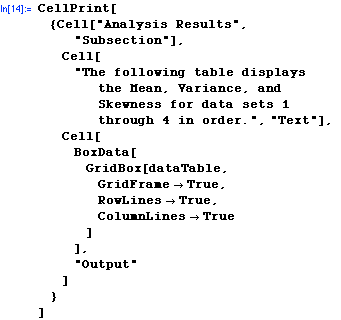``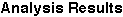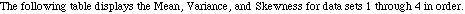## A Simple Prototype of an Automatically Generated Report

The following, when executed, creates a new notebook that has a heading cell followed by a cell with explanatory text and then a cell with the above table. This is therefore a simple example of automatic report generation. (NotebookPut creates the notebook that is defined by the Notebook object which itself contains a list of Cells.)

``````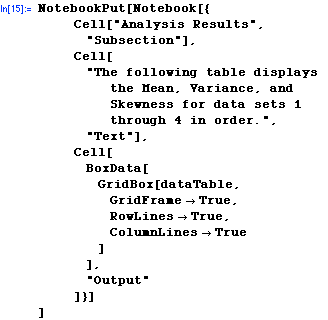``````
`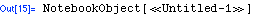`

We are surpressing the display of the generated notbook here, it is displayed (as the output of the function DataReport defined below) at the bottom of this page.

In fact, we can combine the steps that we used above to create a function that automatically generates a report from a data file. The function requires both the function DataStatistics that we defined above as well as the standard package Statistics`DescriptiveStatistics`.

``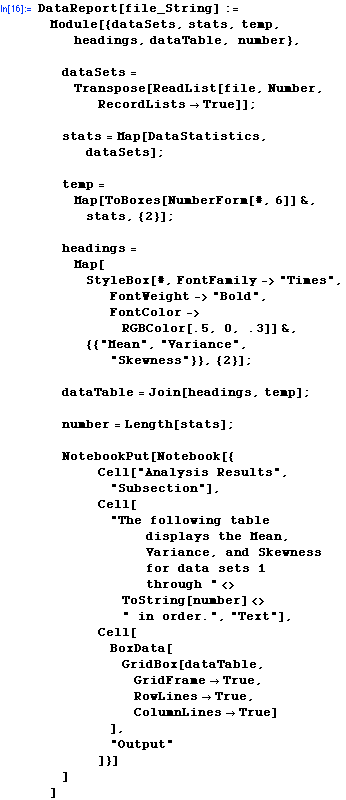``

Here is the report.

``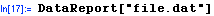``
```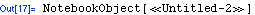```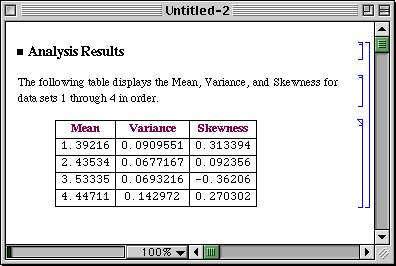For further information on our services send email to info@scientificarts.com . Contents of this web site Copyright © 1999-2011, Scientific Arts, LLC.
 s